### You failed to protect your castle from the powers that be.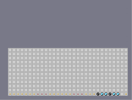Hover over the thumbnail for a full-size version.

Author FusionCoil author:fusioncoil movie rated 2010-05-07 2010-05-07 5 by 10 people. \$You failed to protect your castle from the powers that be.#FusionCoil#movie#11111111111111111111111111111111110000000000001111111111100000000000011111111111000000000000111111111110000000000001111111111100000000000011111111111000000000000111111111110000000000001111111111100000000000011111111111000000000000111111111110000000000001111111111100000000000011111111111000000000000111111111110000000000001111111111100000000000011111111111000000000000111111111110000000000001111111111100000000000011111111111000000000000111111111110000000000001111111111100000000000011111111111000000000000111111111110000000000001111111111100000000000011111111111000000000000111111111110000000000001111111111100000000000011111111111000000000000111111111110000000000001111111111100000000000011111111111000000000000|1^60,564!1^60,540!1^60,516!1^60,492!1^60,468!1^60,444!1^60,420!1^60,396!1^60,372!1^60,348!1^60,324!1^60,300!1^84,300!1^84,324!1^84,348!1^84,372!1^84,396!1^84,420!1^84,444!1^84,468!1^84,492!1^84,516!1^84,540!1^84,564!1^108,564!1^108,540!1^108,516!1^108,492!1^108,468!1^108,444!1^108,420!1^108,396!1^108,372!1^108,348!1^108,324!1^108,300!1^132,300!1^132,324!1^132,348!1^132,372!1^132,396!1^132,420!1^132,444!1^132,468!1^132,492!1^132,516!1^132,540!1^132,564!1^156,564!1^156,540!1^156,516!1^156,492!1^156,468!1^156,444!1^156,420!1^156,396!1^156,372!1^156,348!1^156,324!1^156,300!1^180,300!1^180,324!1^180,348!1^180,372!1^180,396!1^180,420!1^180,444!1^180,468!1^180,492!1^180,516!1^180,540!1^180,564!1^204,564!1^204,540!1^204,516!1^204,492!1^204,468!1^204,444!1^204,420!1^204,396!1^204,372!1^204,348!1^204,324!1^204,300!1^228,300!1^228,324!1^228,348!1^228,372!1^228,396!1^228,420!1^228,444!1^228,468!1^228,492!1^228,516!1^228,540!1^228,564!1^252,540!1^252,516!1^252,492!1^252,468!1^252,444!1^252,420!1^252,396!1^252,372!1^252,348!1^252,324!1^252,300!1^276,300!1^276,324!1^276,348!1^276,372!1^276,396!1^276,420!1^276,444!1^276,468!1^276,492!1^276,516!1^276,540!1^276,564!1^300,564!1^300,540!1^252,564!1^300,516!1^300,492!1^300,468!1^300,444!1^300,420!1^300,396!1^300,372!1^300,348!1^300,324!1^300,300!1^324,300!1^324,324!1^324,348!1^324,372!1^324,396!1^324,420!1^324,444!1^324,468!1^324,492!1^324,516!1^324,540!1^324,564!1^348,564!1^348,540!1^348,516!1^348,492!1^348,468!1^348,444!1^348,420!1^348,396!1^348,372!1^348,348!1^348,324!1^348,300!1^372,564!1^372,540!1^372,516!1^372,492!1^372,468!1^372,444!1^372,420!1^372,396!1^372,372!1^372,348!1^372,324!1^372,300!1^396,300!1^396,324!1^396,348!1^396,372!1^396,396!1^396,420!1^396,444!1^396,468!1^396,492!1^396,516!1^396,540!1^396,564!1^420,564!1^420,540!1^420,516!1^420,492!1^420,468!1^420,444!1^420,420!1^420,396!1^420,372!1^420,348!1^420,324!1^420,300!1^444,300!1^444,324!1^444,348!1^444,372!1^444,396!1^444,420!1^444,444!1^444,468!1^444,492!1^444,516!1^444,540!1^444,564!1^468,564!1^468,540!1^468,516!1^468,492!1^468,468!1^468,444!1^468,420!1^468,396!1^468,372!1^468,348!1^468,324!1^468,300!1^492,300!1^492,324!1^492,348!1^492,372!1^492,396!1^492,420!1^492,444!1^492,468!1^492,492!1^492,516!1^492,540!1^492,564!1^516,564!1^516,540!1^516,516!1^516,492!1^516,468!1^516,444!1^516,420!1^516,396!1^516,372!1^516,348!1^516,324!1^516,300!1^540,300!1^540,324!1^540,348!1^540,372!1^540,396!1^540,420!1^540,444!1^540,468!1^540,492!1^540,516!1^540,540!1^540,564!1^564,564!1^564,540!1^564,516!1^564,492!1^564,468!1^564,444!1^564,420!1^564,396!1^564,372!1^564,348!1^564,324!1^564,300!1^588,564!1^612,564!1^636,564!1^660,564!1^684,564!1^708,564!1^732,564!1^756,564!1^756,540!1^732,540!1^708,540!1^684,540!1^660,540!1^636,540!1^612,540!1^588,540!1^588,516!1^612,516!1^636,516!1^660,516!1^684,516!1^708,516!1^732,516!1^756,516!1^756,492!1^732,492!1^708,492!1^684,492!1^660,492!1^636,492!1^612,492!1^588,492!1^588,468!1^612,468!1^636,468!1^660,468!1^684,468!1^708,468!1^732,468!1^756,468!1^756,444!1^732,444!1^708,444!1^684,444!1^660,444!1^636,444!1^612,444!1^588,444!1^588,420!1^612,420!1^636,420!1^660,420!1^684,420!1^708,420!1^732,420!1^756,420!1^756,396!1^756,372!1^756,348!1^756,324!1^756,300!1^588,396!1^588,372!1^612,396!1^612,372!1^612,348!1^612,324!1^636,396!1^636,372!1^636,348!1^660,396!1^684,396!1^684,372!1^708,396!1^708,372!1^708,348!1^708,324!1^708,300!1^732,348!1^732,372!1^732,396!1^660,372!1^660,348!1^684,348!1^588,348!1^588,324!1^588,300!1^612,300!1^636,324!1^636,300!1^660,300!1^660,324!1^684,324!1^684,300!1^732,300!1^732,324!6^588,564,5,0,2,1!6^588,540,5,0,2,1!6^588,516,5,0,2,1!6^588,492,5,0,2,1!6^588,468,5,0,2,1!6^588,444,5,0,2,1!6^588,420,5,0,2,1!6^564,396,5,0,2,1!6^588,396,5,0,2,1!6^612,396,5,0,2,1!6^612,372,5,0,2,1!6^564,372,5,0,2,1!6^732,564,5,0,2,1!6^732,540,5,0,2,1!6^732,516,5,0,2,1!6^732,492,5,0,2,1!6^732,468,5,0,2,1!6^732,444,5,0,2,1!6^732,420,5,0,2,1!6^732,396,5,0,2,1!6^708,396,5,0,2,1!6^708,372,5,0,2,1!6^756,372,5,0,2,1!6^756,396,5,0,2,1!6^660,564,5,0,2,1!6^660,540,5,0,2,1!6^612,468,5,0,0,1!6^636,444,5,0,0,1!6^660,468,5,0,0,1!6^684,444,5,0,0,1!6^708,468,5,0,0,1!6^636,468,5,0,0,1!6^684,468,5,0,0,1!6^708,492,5,0,0,1!6^684,492,5,0,0,1!6^660,492,5,0,0,1!6^636,492,5,0,0,1!6^612,492,5,0,0,1!6^612,516,5,0,0,1!6^636,516,5,0,0,1!6^660,516,5,0,0,1!6^684,516,5,0,0,1!6^708,516,5,0,0,1!6^708,540,5,0,0,1!6^684,540,5,0,0,1!6^636,540,5,0,0,1!6^612,540,5,0,0,1!6^612,564,5,0,0,1!6^636,564,5,0,0,1!6^684,564,5,0,0,1!6^708,564,5,0,0,1!12^588,372!12^588,348!12^588,324!0^564,324!12^732,372!12^732,348!12^732,324!0^708,324!0^60,564!0^84,564!0^108,564!0^132,564!0^156,564!0^180,564!0^204,564!0^228,564!0^252,564!0^276,564!0^300,564!0^324,564!0^348,564!0^372,564!0^396,564!0^420,564!0^444,564!0^468,564!0^492,564!0^516,564!0^540,564!0^564,564!0^756,564!1^444,468!1^444,468!1^444,468!1^444,468!1^444,468!1^444,468!1^444,468!1^444,468!1^444,468!1^444,468!1^444,468!1^444,468!1^444,468!1^444,468!1^444,468!1^444,468!1^444,468!1^444,468!1^444,468!1^444,468!1^444,468!1^444,468!1^444,468!1^444,468!1^444,468!1^444,468!1^444,468!1^444,468!1^444,468!1^444,468!1^444,468!1^444,468!1^444,468!1^444,468!1^444,468!1^444,468!1^444,468!6^60,540,5,0,2,1!1^132,492!1^132,492!1^132,492!1^132,492!1^132,492!6^84,540,5,0,2,1!12^60,516!12^60,492!1^132,516!1^132,516!1^132,516!1^132,516!1^132,516!6^108,540,5,0,2,1!12^84,516!12^84,492!1^156,492!1^156,492!1^156,492!1^156,492!1^156,492!6^132,540,5,0,2,1!1^60,516!1^60,492!12^108,492!12^108,516!1^204,492!1^204,492!1^204,492!1^204,492!1^204,492!1^60,540!6^156,540,5,0,2,1!1^84,516!1^84,492!12^132,492!12^132,516!1^228,516!1^228,516!1^228,516!1^228,516!1^228,516!1^84,540!6^180,540,5,0,2,1!1^108,492!1^108,516!12^156,492!12^156,516!1^252,492!1^252,492!1^252,492!1^252,492!1^252,492!1^252,492!1^252,492!1^252,492!1^252,492!1^252,492!1^252,492!1^252,492!1^252,492!1^252,492!1^252,492!1^252,492!1^252,492!1^252,492!1^252,492!1^252,492!0^180,492!1^204,492!1^204,492!1^204,492!1^204,492!1^204,492!0^204,492!1^228,492!1^228,492!1^228,492!1^228,492!1^228,492!0^228,492!1^252,492!1^252,492!1^252,492!1^252,492!1^252,492!0^228,516!0^228,468!1^372,492!1^372,492!1^372,492!1^372,492!1^372,492!1^372,492!1^372,492!1^372,492!1^372,492!1^372,492!1^372,492!1^372,492!1^372,492!1^372,492!1^372,492!1^372,492!1^372,492!1^372,492!1^372,492!1^372,492!1^372,492!1^372,492!1^372,492!1^372,492!1^372,492!1^372,492!1^372,492!6^252,492,2,0,1,0!1^276,468!1^276,468!1^276,468!1^276,468!1^276,468!1^276,468!1^252,492!6^276,492,2,0,1,0!1^276,468!1^276,468!1^276,468!1^276,468!1^276,468!1^276,468!1^276,492!6^300,492,2,0,1,0!1^300,468!1^300,468!1^300,468!1^300,468!1^300,468!1^300,468!1^300,492!6^324,492,2,0,1,0!1^324,468!1^324,468!1^324,468!1^324,468!1^324,468!1^324,468!0^252,300!1^372,468!1^372,468!1^372,468!1^372,468!1^372,468!1^372,468!1^324,492!6^348,492,2,0,1,0!0^276,300!0^228,300!0^252,324!1^300,324!1^300,324!1^300,324!1^300,324!1^300,324!1^300,324!1^348,492!6^372,492,2,0,1,0!1^228,300!1^252,300!0^276,324!0^276,348!0^300,324!12^252,300!12^228,324!12^228,300!1^348,348!1^348,348!1^348,348!1^348,348!1^348,348!1^348,348!1^372,492!6^396,492,2,0,1,0!1^276,324!1^252,324!1^276,300!0^300,348!0^324,348!0^300,372!12^252,348!12^276,324!12^252,324!1^372,396!1^372,396!1^372,396!1^372,396!1^372,396!1^396,492!6^420,492,2,0,1,0!1^276,348!1^300,348!1^300,324!0^324,372!0^324,396!0^348,372!12^276,372!12^276,348!12^300,348!1^444,468!1^444,468!1^444,468!1^444,468!1^444,468!1^444,468!1^420,492!6^444,492,2,0,1,0!1^396,420!1^396,420!1^396,420!1^396,420!1^396,420!1^396,420!1^300,372!1^324,348!1^324,372!0^348,396!0^348,420!0^372,396!12^324,372!12^300,396!12^300,372!1^420,444!1^420,444!1^420,444!1^420,444!1^420,444!1^420,444!1^324,396!1^348,372!1^348,396!0^372,420!0^372,444!0^396,420!12^324,396!12^324,420!12^348,396!1^348,420!1^372,396!1^372,420!0^396,444!0^396,468!0^420,444!12^348,444!12^348,420!12^372,420!1^372,396!1^372,396!1^372,396!1^372,396!1^372,396!1^372,396!1^372,444!1^396,444!1^396,420!0^420,468!0^420,492!0^444,468!12^372,468!12^372,444!12^396,444!1^444,444!1^444,444!1^444,444!1^444,444!1^444,444!1^444,444!1^396,468!1^420,468!1^420,444!1^444,492!0^444,492!0^444,516!0^468,492!12^396,492!12^396,468!12^420,468!1^420,444!1^420,444!1^420,444!1^420,444!1^420,444!1^420,444!1^420,492!1^444,492!1^444,468!0^468,516!0^468,540!0^492,516!12^420,516!12^420,492!12^444,492!1^468,468!1^468,468!1^468,468!1^468,468!1^468,468!1^468,468!1^444,516!1^468,516!1^468,492!0^492,540!0^516,540!12^444,540!12^444,516!12^468,516!1^468,444!1^468,444!1^468,444!1^468,444!1^468,444!1^468,444!1^228,324!1^228,300!1^252,300!1^252,348!1^252,324!1^276,324!1^492,516!1^444,444!1^444,444!1^444,444!1^444,444!1^444,444!1^444,444!1^276,372!1^276,348!1^300,348!1^300,396!1^300,372!1^324,372!1^468,540!1^492,540!1^516,540!12^468,468!12^516,516!12^540,468!1^516,444!1^516,444!1^516,444!1^516,444!1^516,444!1^516,444!1^324,420!1^324,396!1^348,396!12^444,420!12^372,540!1^492,564!1^492,564!1^492,564!1^492,564!12^492,564!1^468,564!12^468,564!12^492,420!12^324,516!1^324,420!1^324,420!1^324,420!1^324,420!1^324,420!1^324,420!1^348,444!1^348,420!1^372,420!1^372,468!1^372,444!1^396,444!12^348,468!12^372,396!12^324,420!1^420,372!1^420,372!1^420,372!1^420,372!1^420,372!1^420,372!1^444,564!12^444,564!1^396,492!1^396,468!1^420,468!1^420,516!1^420,492!1^444,492!12^396,516!12^420,444!12^372,444!1^444,492!1^444,492!1^444,492!1^444,492!1^444,492!1^444,492!1^444,540!1^444,516!12^492,372!12^420,492!1^420,396!1^420,396!1^420,396!1^420,396!1^420,396!1^420,396!1^324,420!1^372,396!1^372,444!1^372,420!1^372,420!1^372,420!1^372,420!1^372,420!1^372,420!1^492,372!1^444,420!1^492,420!1^468,396!1^468,396!1^468,396!1^468,396!1^468,396!1^468,396!1^324,516!1^348,468!1^420,444!1^420,420!1^420,420!1^420,420!1^420,420!1^420,420!1^420,420!1^468,468!1^540,468!1^516,516!0^444,300!1^468,324!1^468,324!1^468,324!1^468,324!1^468,324!1^468,324!1^396,516!1^420,492!1^468,516!0^444,324!0^420,300!0^468,300!1^372,540!1^372,540!1^372,540!1^372,540!1^372,540!1^372,540!1^420,300!1^468,300!0^420,324!0^468,324!12^468,300!1^468,348!1^468,348!1^468,348!1^468,348!1^468,348!1^468,348!1^444,324!1^444,300!1^468,324!0^396,348!0^420,348!0^420,372!12^444,300!12^444,324!12^468,324!1^468,348!1^468,348!1^468,348!1^468,348!1^468,348!1^468,348!1^420,324!1^420,348!0^372,372!0^396,372!0^396,396!12^420,324!12^420,348!12^444,348!1^468,348!1^468,348!1^468,348!1^468,348!1^468,348!1^468,348!1^468,372!1^468,372!1^468,372!1^468,372!1^468,372!1^468,372!1^396,348!1^396,372!1^420,372!0^348,396!0^372,396!0^372,420!12^396,348!12^396,372!12^420,372!1^444,396!1^444,396!1^444,396!1^444,396!1^444,396!1^444,396!1^372,372!1^372,396!1^396,396!0^324,420!0^348,420!0^348,444!12^372,372!12^372,396!12^396,396!1^420,396!1^420,396!1^420,396!1^420,396!1^420,396!1^420,396!1^348,396!1^348,420!1^372,420!0^300,444!0^324,444!0^324,468!12^348,396!12^348,420!12^372,420!1^396,420!1^396,420!1^396,420!1^396,420!1^396,420!1^396,420!1^324,420!1^324,444!1^348,444!0^276,468!0^300,468!0^300,492!12^324,420!12^324,444!12^348,444!1^372,444!1^372,444!1^372,444!1^372,444!1^372,444!1^372,444!1^300,444!1^300,468!1^324,468!0^252,492!0^276,492!0^276,516!12^300,444!12^300,468!12^324,468!1^348,468!1^348,468!1^348,468!1^348,468!1^348,468!1^348,468!1^276,468!1^276,492!1^300,492!0^252,516!0^252,540!12^276,468!12^276,492!12^300,492!1^324,492!1^324,492!1^324,492!1^324,492!1^324,492!1^324,492!1^228,516!1^252,492!1^276,516!0^228,540!0^276,540!12^252,492!12^276,516!1^300,516!1^300,516!1^300,516!1^300,516!1^300,516!1^300,516!1^252,516!1^228,540!1^252,540!12^252,516!12^228,540!12^252,540!1^324,516!1^324,516!1^324,516!1^324,516!1^324,516!1^324,516!1^444,300!1^468,300!1^468,324!1^444,348!1^444,324!1^420,324!1^276,540!12^324,540!12^372,492!12^252,444!1^252,420!1^252,420!1^252,420!1^252,420!1^252,420!1^252,420!1^396,348!1^420,348!1^420,372!1^396,396!1^396,372!1^372,372!12^204,420!12^252,372!12^396,468!12^372,540!1^252,564!1^228,564!12^228,564!12^252,564!1^276,564!1^276,564!1^276,564!1^276,564!1^276,564!1^276,564!12^276,564!12^204,516!1^348,396!1^372,396!1^372,420!1^348,444!1^348,420!1^324,420!1^228,492!1^228,468!1^204,492!1^180,492!12^180,468!12^276,396!1^372,444!1^372,444!1^372,444!1^372,444!1^372,444!1^372,444!1^300,444!1^324,444!1^324,468!1^276,468!1^300,468!1^300,492!0^84,492!0^108,420!0^228,492!1^228,468!1^228,468!1^228,468!1^228,468!1^228,468!1^228,468!0^132,444!0^156,372!0^180,444!0^228,396!0^60,444!1^156,420!1^156,420!1^156,420!1^156,420!1^156,420!1^156,420!0^180,492!0^108,372!0^204,348!1^276,468!1^276,468!1^276,468!1^276,468!1^276,468!1^276,468!1^252,492!1^276,492!1^276,516!1^132,492!1^156,492!1^156,516!12^132,492!12^84,444!12^156,396!1^180,396!1^180,396!1^180,396!1^180,396!1^180,396!1^180,396!12^204,444!12^132,348!12^108,492!12^84,396!1^180,516!1^180,516!1^180,516!1^180,516!1^180,516!1^180,516!1^132,516!1^108,492!1^180,540!12^156,324!12^276,468!12^60,540!1^252,516!1^252,516!1^252,540!1^252,540!1^252,540!1^228,540!1^156,540!1^132,540!12^276,516!12^84,348!0^156,540!0^60,396!0^180,300!1^228,420!1^228,420!1^228,420!1^228,420!1^108,540!1^396,468!1^372,492!1^372,492!1^372,492!1^372,492!1^372,492!1^372,492!1^372,492!1^180,300!1^156,324!1^204,348!1^204,348!1^204,348!1^204,348!1^204,348!1^204,348!1^204,348!1^84,348!1^132,348!1^108,372!1^132,348!1^132,348!1^132,348!1^132,348!1^132,348!1^132,348!1^60,396!1^84,396!1^108,420!1^108,396!1^108,396!1^108,396!1^108,396!1^108,396!1^108,396!1^156,396!1^156,372!1^228,396!1^276,372!1^276,372!1^276,372!1^276,372!1^276,372!1^276,372!1^252,372!1^276,396!1^204,420!1^204,444!1^60,444!1^84,444!1^108,444!1^108,444!1^108,444!1^108,444!1^108,444!1^108,444!1^132,444!1^180,468!1^180,444!1^252,444!1^252,444!1^252,444!1^252,444!1^252,444!1^252,444!1^252,444!1^372,540!1^324,540!1^276,468!1^276,516!1^156,492!1^156,492!1^156,492!1^156,492!1^156,492!1^156,492!1^60,540!1^84,492!1^132,492!1^204,516!1^204,492!1^204,492!1^228,492!1^180,492!1^156,540!1^156,540!1^156,540!1^156,540!1^156,540!1^156,540!1^156,540!1^156,540!1^156,540!1^156,540!1^348,492!1^348,492!1^348,492!1^348,492!1^348,492!1^348,492!1^348,492!1^348,492!1^348,492!1^348,492!1^348,492!1^348,492!1^348,492!1^348,492!1^348,492!1^348,492!1^348,492!1^348,492!1^348,492!1^348,492!0^348,300!1^396,300!1^396,300!1^396,300!1^396,300!1^396,300!0^324,300!0^348,324!0^372,300!1^396,300!1^396,300!1^396,300!1^396,300!1^396,300!1^324,300!1^372,300!0^372,324!0^324,324!0^348,348!12^324,300!1^396,324!1^396,324!1^396,324!1^396,324!1^396,324!1^396,324!1^348,300!1^324,324!1^348,324!0^372,348!0^372,372!0^396,348!12^324,324!12^348,324!12^348,300!1^396,300!1^396,300!1^396,300!1^396,300!1^396,300!1^396,300!1^348,348!1^372,348!1^372,324!0^396,372!0^396,396!0^420,372!12^348,348!12^372,348!12^372,324!1^420,324!1^420,324!1^420,324!1^420,324!1^420,324!1^372,372!1^396,372!1^396,348!0^420,396!0^420,420!0^444,396!12^372,372!12^396,372!12^396,348!1^420,348!1^420,348!1^420,348!1^420,348!1^420,348!1^396,396!1^420,396!1^420,372!0^444,420!0^444,444!0^468,420!12^396,396!12^420,396!12^420,372!1^492,420!1^492,420!1^492,420!1^492,420!1^420,420!1^444,420!1^444,396!0^468,444!0^468,468!0^492,444!12^420,420!12^444,420!12^444,396!1^492,396!1^492,396!1^492,396!1^492,396!1^492,396!1^444,444!1^468,444!1^468,420!0^492,468!0^492,492!0^516,468!12^444,444!12^468,444!12^468,420!1^492,420!1^492,420!1^492,420!1^492,420!1^492,420!1^468,468!1^492,468!1^492,444!0^516,492!0^516,516!0^540,492!12^468,468!12^492,468!12^492,444!1^516,444!1^516,444!1^516,444!1^516,444!1^516,444!1^492,492!1^516,492!1^516,468!0^540,516!0^540,540!0^564,516!12^492,492!12^516,492!12^516,468!1^540,468!1^540,468!1^540,468!1^540,468!1^540,468!1^516,516!1^540,516!1^540,492!0^564,540!12^516,516!12^540,516!12^540,492!1^516,444!1^516,444!1^516,444!1^516,444!1^516,444!1^516,444!1^324,324!1^324,300!1^348,300!1^540,540!1^588,516!1^588,540!1^612,516!12^588,516!12^612,516!12^588,540!12^564,468!1^564,516!1^564,516!1^564,516!1^564,516!1^564,516!1^564,516!1^348,348!1^348,324!1^372,324!1^372,372!1^372,348!1^396,348!1^588,444!1^636,492!1^588,492!1^612,492!12^492,540!12^516,420!12^636,420!12^540,372!1^468,372!1^468,372!1^468,372!1^468,372!1^468,372!1^396,396!1^396,372!1^420,372!1^420,420!1^420,396!1^444,396!1^564,372!1^588,396!1^612,468!1^588,420!12^588,444!12^492,372!12^420,540!12^372,468!1^564,444!1^564,444!1^564,444!1^564,444!1^564,444!0^420,492!0^420,372!0^564,444!0^516,324!0^468,540!1^588,492!1^588,492!1^588,492!1^588,492!1^588,492!1^612,540!1^636,516!1^636,444!1^564,396!1^612,396!1^612,372!1^612,396!1^612,396!1^612,396!1^612,396!1^612,396!1^588,348!1^588,324!12^372,540!12^372,396!12^468,324!12^636,348!1^636,444!1^636,444!1^636,444!1^636,444!1^636,444!1^588,468!1^444,444!1^444,420!1^468,420!1^468,468!1^468,444!1^492,444!1^660,516!1^636,540!1^660,540!1^660,492!12^636,492!12^588,396!12^444,444!12^468,396!12^444,516!1^660,420!1^660,420!1^660,420!1^660,420!1^660,420!1^660,468!1^684,444!1^708,468!1^684,492!1^684,516!1^684,468!12^684,516!12^660,444!12^708,420!12^684,468!1^684,444!1^684,444!1^684,444!1^684,444!1^684,444!0^636,540!0^660,372!0^612,468!0^468,492!0^612,324!1^684,348!1^684,348!1^684,348!1^684,348!1^684,348!1^708,372!1^732,348!1^732,324!1^732,348!1^732,348!1^732,348!1^732,348!1^732,348!1^732,564!1^732,540!1^708,468!1^708,468!1^708,468!1^708,468!1^708,468!1^708,396!1^732,396!1^756,396!1^756,372!1^756,492!1^756,492!1^756,492!1^756,492!1^756,492!12^660,324!12^756,300!12^756,420!12^324,516!1^540,420!1^540,420!1^540,420!1^540,420!1^540,420!1^732,420!1^732,444!1^732,468!1^708,492!1^732,492!1^732,516!1^708,516!1^708,516!1^708,516!1^708,516!1^708,516!1^708,516!1^708,540!1^684,540!12^732,516!12^732,468!12^756,540!12^396,348!12^348,492!1^564,420!1^564,420!1^564,420!1^564,420!1^564,420!0^684,420!0^660,516!0^708,540!0^756,372!0^684,300!1^348,372!1^348,372!1^348,372!1^348,372!1^348,372!1^324,516!1^348,492!1^372,468!1^444,444!1^492,492!1^492,468!1^516,468!1^516,492!1^516,492!1^516,492!1^516,492!1^516,492!1^540,492!1^540,516!1^516,516!1^516,420!1^492,372!1^492,372!1^492,372!1^492,372!1^492,372!1^468,324!1^420,372!1^396,348!1^372,396!1^420,396!1^420,396!1^420,396!1^420,396!1^420,396!1^372,540!1^420,492!1^420,540!1^444,516!1^564,396!1^564,396!1^564,396!1^564,396!1^564,396!1^756,300!1^708,324!1^684,300!1^660,324!1^660,324!1^660,324!1^660,324!1^660,324!1^612,324!1^564,324!1^516,324!1^468,396!1^468,396!1^468,396!1^468,396!1^468,396!1^468,396!1^468,540!1^468,492!1^492,540!1^564,540!1^564,516!1^564,516!1^564,516!1^564,516!1^564,516!1^540,372!1^588,372!1^636,348!1^732,372!1^732,372!1^732,372!1^732,372!1^732,372!1^732,372!1^756,372!1^660,372!1^588,396!1^636,420!1^636,420!1^636,420!1^636,420!1^636,420!1^636,420!1^588,444!1^564,468!1^564,444!1^708,420!1^708,420!1^708,420!1^708,420!1^708,420!1^708,420!1^684,420!1^756,420!1^732,468!1^684,468!1^684,468!1^684,468!1^684,468!1^684,468!1^684,468!1^660,444!1^612,468!1^636,492!1^612,516!1^612,516!1^612,516!1^612,516!1^612,516!1^612,516!1^588,516!1^588,540!1^636,540!1^660,516!1^636,468!1^684,516!1^684,516!1^684,516!1^684,516!1^684,516!1^732,516!1^708,540!1^756,540!1^516,444!1^516,444!1^516,444!1^516,444!1^516,444# Game over. Epic fail. Go rebuild :) My first movie in ages. Took some time. Watch it load. You know you want to. Njoy! :D

## Other maps by this author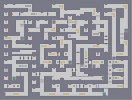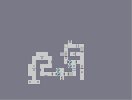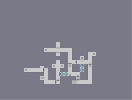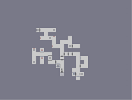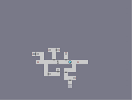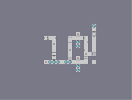The Invalid Phase. Fusion's sixth with some new items map. Fusion's seventh with a seeking zap drone. Fusion's eight with no zap drones... Fusion's ninth with only one drone on the playable bit. Fusion's tenth aniversary edition.

Pages: (0)

me wanna go YES

### I don't like movies

because most are bad, but this one rocks! 5aved

### Completely agree with Pretzel.

4/5

Its a little dodgey, but I know how hard they are, and its good to see you making one of these again.

### demo!

Demo Data 0:0

this is awesome

### ooh a movie!

i love these! pretty cool 5

### uh, troopa, most good /maps/ take hours

A lot of the best mappers spend weeks on their maps. So yeah. :p

### ThAT IS AWESOME!

5/5 must have taken hours!!!